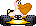#Phil’s Wacky Wheels SiteSkip navigation

## Editing time trial scores

`WACKY1.TIM` contains the best times for time trials with 12 hp engines. `WACKY2.TIM` contains times for 6 hp engines. Each file contains 30 lines, each 120 bytes long (but there are no line breaks in the file). Each line contains the following:

• At the start of each line are the four names of the drivers with best single lap times. Each name is 16 bytes long, followed by four NUL bytes (ASCII 00). The names can contain upper case letters, numbers, spaces, or these characters: `! # & ( ) - , . ' ?`
• After the four names (each followed by four NUL bytes), there are four longints (four bytes each). The value of the longint is the time in seconds, multiplied by ten. The maximum time allowed is 60 minutes, or a longint value of 36,000. Because Wacky Wheels won’t recognize times over 60 minutes, the last two bytes of each longint will always be 00. Information on how to read or edit the first two bytes is below.
• The next 16 bytes contain the overall best time for the entire race. The next four bytes are NUL.
• The next four bytes are a longint from 0 to 36,000 containing the best overall race time. After that, the next line starts.

Here’s how to edit the two bytes containing the time:

### Convert the two bytes into a time

• Divide the value of the first byte by ten.
• Multiply the value of the second byte by 25.6.
• Add these numbers and you have the time in seconds.

For example, if the two bytes read “60 1” (in decimal, or “3C 01” in hex), the time is (60 / 10) + (1 * 25.6) = 31.6.

### Find the bytes needed for a particular time

• Divide the time in seconds by 25.6.
• The number to the left of the decimal is the value of the second byte.
• Drop everything left of the decimal (leaving the fractional part). Multiply this by 256, and you have the value of the first byte.

For example, a time of 1 minute, 39.6 seconds equals 99.6 seconds. 99.6 / 25.6 = 3.890625. 0.890625 * 256 = 228, so the two bytes should be “228 3.”

For programming computers, you can convert a time into two bytes this way:

• First byte = (time in seconds * 10) % 256. (time % 25.6 will result in rounding errors.)
• Second byte: floor(time * 10 / 256)